﻿ 一种新型旋涡发生体的数值模拟与研究

# 一种新型旋涡发生体的数值模拟与研究Numerical Simulation and Study of a New Type Vortex Shedder

Abstract: Vortex shedder is an important component of vortex flowmeter. Its shape has great influence on the measurement range, measurement precision and vortex intensity of vortex flowmeter. In this paper, the flow field of a new type vortex shedder is numerically simulated by Fluent. The new vortex shedder is compared with a commonly used trapezoidal vortex shedder on the stability of Strouhal number (stability of vortex-shedding), vortex strength and irrecoverable pressure loss. The results show that the Strouhal number of the new type vortex shedder can maintain a constant within the larger Reynolds number range, and the stability of Strouhal number is better than that of the trapezoidal vortex shedder. The vortex intensity generated by the new type vortex shedder is much greater than that of the trapezoidal vortex shedder. Within the Reynolds number Re < 199040, the irrecoverable pressure loss of the two vortex shedder is almost the same.

1. 引言

2. 涡街流量计的结构与测量原理

$f=St\frac{u}{md}$ (1)

$m=1-\frac{2}{\pi }\left[\frac{d}{D}\sqrt{1-{\left(\frac{d}{D}\right)}^{2}}+\mathrm{arcsin}\left(\frac{d}{D}\right)\right]$ (2)

${q}_{v}=\frac{\pi {D}^{2}u}{4}=\frac{\pi {D}^{2}mdf}{4St}=\frac{f}{K}$ (3)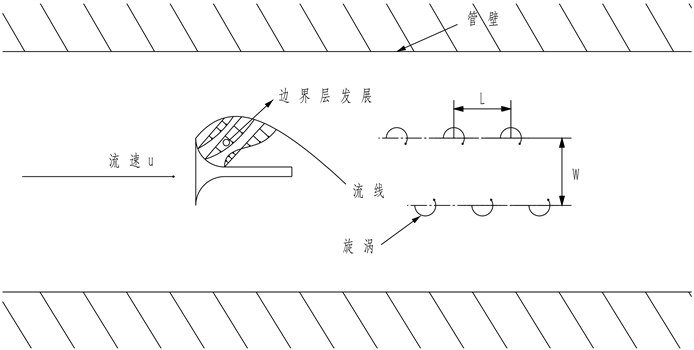Figure 1. The sketch map of Kaman vortex street

3. 计算模型与模拟结果分析

3.1. 新型旋涡发生体结构

3.2. 计算模型

4. 流场的模拟结果与分析

4.1. 两种漩涡发生体的涡量云图和压力云图

4.2. 压力波动频率及斯特劳哈尔数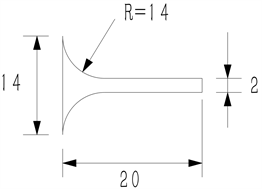(a) 新型旋涡发生体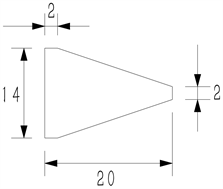(b) 梯形旋涡发生体

Figure 2. The structure of two kinds of vortexes shedder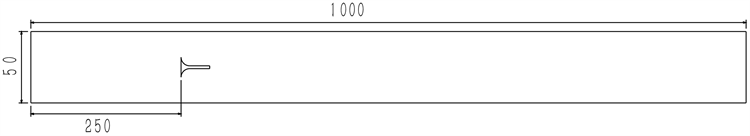Figure 3. The calculation area of the new type of vortex shedder’s flow field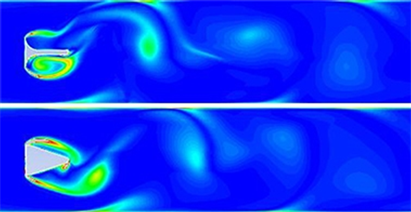Figure 4. The vorticity cloud graph of the new type of vortex shedder and trapezoidal vortex shedder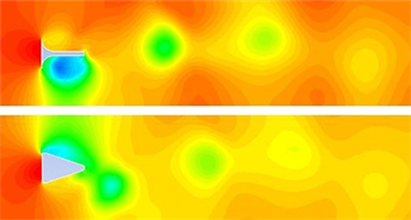Figure 5. The pressure cloud graph of the new type of vortex shedder and trapezoidal vortex shedder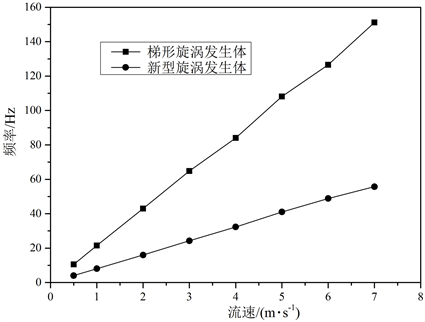Figure 6. The change relationship of the frequencies with the Reynolds number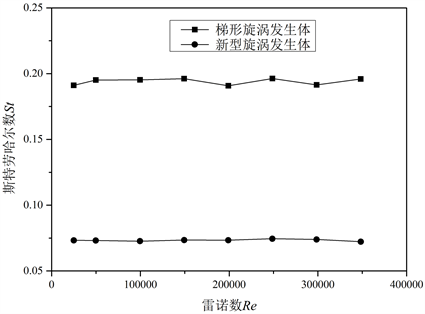Figure 7. The change relationship of the strouhal number with the Reynolds number

$\epsilon =\frac{1}{N}\underset{i=1}{\overset{N}{\sum }}\frac{|S{t}_{i}-\stackrel{¯}{St}|}{\stackrel{¯}{St}}×100%$ (4)

Sti—不同雷诺数下的斯特劳哈尔数；

$\stackrel{¯}{St}$ —平均斯特劳哈尔数；

N——数据点数。

4.3. 旋涡强度的比较

4.4. 永久压降的比较Figure 8. The pressure fluctuation curve of the monitoring point of the trapezoidal vortex shedder’s flow field when the Reynolds number is 49760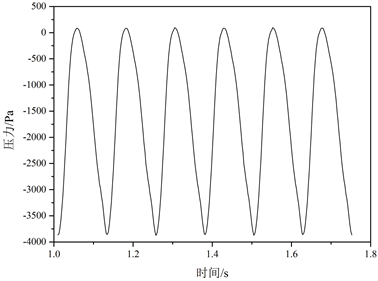Figure 9. The pressure fluctuation curve of the monitoring point of the new type of vortex sheder’s flow field when the Reynolds number is 49760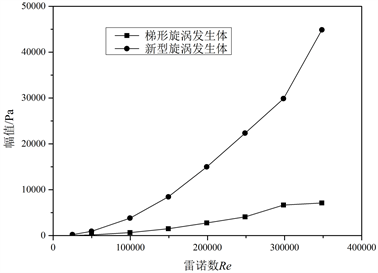Figure 10. The change relationship of the amplitude of pressure fluctuation with the Reynolds number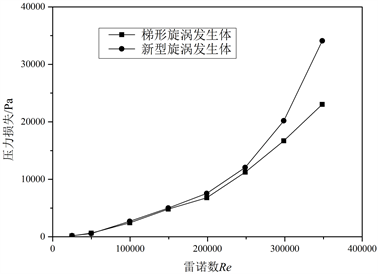Figure 11. The change relationship of the pressure loss with the Reynolds number

5. 结论

1) 通过对两种旋涡发生体的模拟与比较，发现在较大雷诺数范围内，新型旋涡发生体的斯特劳哈尔数都为一个常数(St = 0.07332)。在相同雷诺数范围内，新型旋涡发生体的斯特劳哈尔数稳定性也好于梯形旋涡发生体，即新型旋涡发生体的旋涡脱落更稳定。所以新型旋涡发生体的测量范围更大，测量的稳定性也好于梯形旋涡发生体。

2) 在雷诺数以及流体介质相同的情况下，对两种旋涡发生体监测点处压力波动大小进行了比较，发现新型旋涡发生体所产生的旋涡强度比梯形旋涡发生体的大得多，不仅更利于旋涡信号的检测，并且能够减小干扰的影响以及降低检测的下限。

3) 在雷诺数不是很大的时候，两种旋涡发生体的永久压力损失相差很小。

 Achenbach, E. (2006) Distribution of Local Pressure and Skin Friction around a Circular Cylinder in Cross-Flow up to Re = 5106. Journal of Fluid Mechanics, 34, 625-639.
https://doi.org/10.1017/S0022112068002120

 Miau, J.J., Yang, C.C., Chou, J.H., et al. (1993) A T-Shaped Vortex Shedder for a Vortex Flow Meter. Flow Measurement & Instrumentation, 4, 259-267.
https://doi.org/10.1016/0955-5986(93)90033-F

 Coulthard, J. and Yan, Y. (1993) Comparisons of Different Bluff Bodies in Vortex Wake Transit Time Measurements. Flow Measurement & Instrumentation, 4, 273-275.
https://doi.org/10.1016/0955-5986(93)90035-H

 Igarashi, T. (2008) Flow Resistance and Strouhal Number of a Vortex Shedder in a Circulapipe. JSME International Journal, 42, 586-595.
https://doi.org/10.1299/jsmeb.42.586

 Matsunaga, Y., Takahashi, S. and Kuromori, K. (1990) Numerical Analysis of a Vortex Flowmeter and Comparison with Experiment. Flow Measurement & Instrumentation, 1, 106-112.
https://doi.org/10.1016/0955-5986(90)90033-4

 Wahed, A.K.E., Johnson, M.W. and Sproston, J.L. (1993) Numerical Study of Vortex Shedding from Different Shaped Bluff Bodies. Flow Measurement & Instrumentation, 4, 233-240.
https://doi.org/10.1016/0955-5986(93)90030-M

 贾云飞, 张涛, 邢娟. 基于FLUENT对涡街流量传感器流场仿真及特性研究[J]. 系统仿真学报, 2007, 19(12): 2683-2685.

 Reik, M., Höcker, R., Bruzzese, C., et al. (2010) Flow Rate Measurement in a Pipe Flow by Vortex Shedding. Forschung Im Ingenieurwesen, 74, 77-86.
https://doi.org/10.1007/s10010-010-0117-0

 胡岳, 张涛. 涡街流量计梯形旋涡发生体的研究[J]. 化工自动化及仪表, 2012, 39(5): 580-584.

 Johnson, R., Schniter, P., Endres, T.J., et al. (2002) Blind Equalization Using the Constant Modulus Criterion: A Review. Proceedings of the IEEE, 86, 1927-1950.
https://doi.org/10.1109/5.720246

 Roshko, A. (1961) Experiments on the Flow past a Circular Cylinder at Very High Reynolds Number. Journal of Fluid Mechanics, 10, 345-356.
https://doi.org/10.1017/S0022112061000950

 朱德祥. 流量仪表原理和应用[M]. 上海: 华东化工学院出版社, 1992.

 Venugopal, A., Agrawal, A. and Prabhu, S.V. (2011) Review on Vortex Flowmeter—Designer Perspective. Sensors & Actuators a Physical, 170, 8-23.

Top